Symbol
ProblemFind the domain and range of the following rational function. Use any notation. $1$ $f\left(x\right)=\dfrac {2} {x+1}$ $2$ $f\left(x\right)=\dfrac {3x} {x+3}$ $3.$ $f\left(x\right)=\dfrac {3-x} {x-7}$ $4$ $f\left(x\right)=\dfrac {2+x} {x}$ $5.f\left(x\right)=\dfrac {\left(x+1\right)} {x2-1}$
10th-13th grade
Algebra
Search count: 113
Question content
Anybody who can help me answering these.I really need with solution.
SolutionQanda teacher - Sneha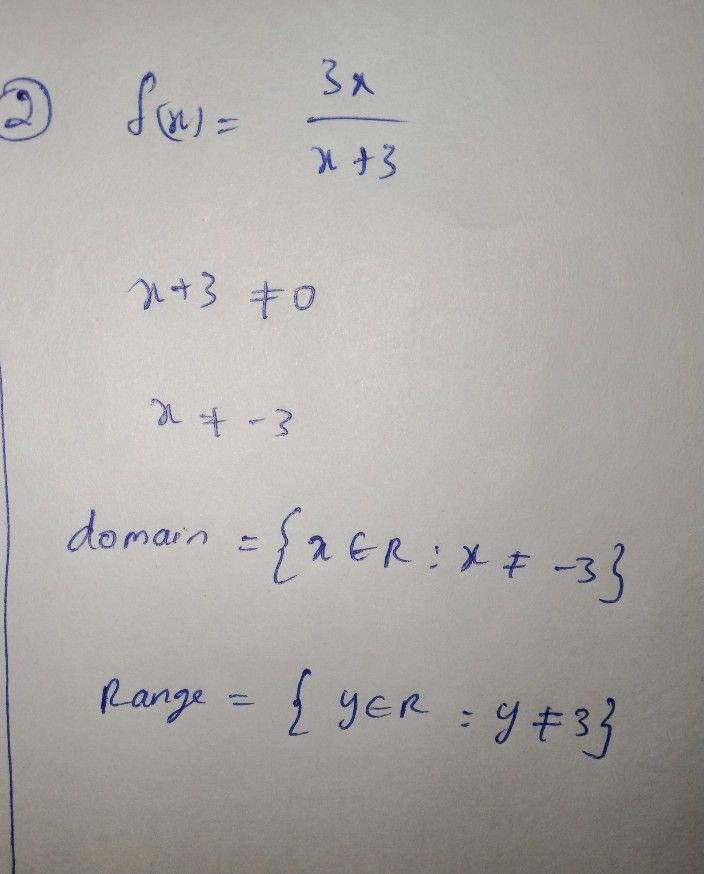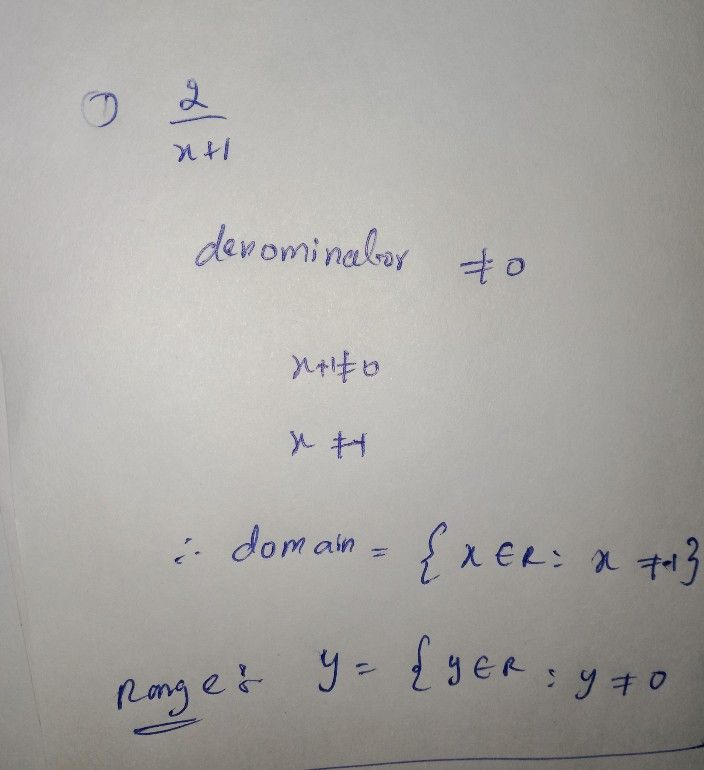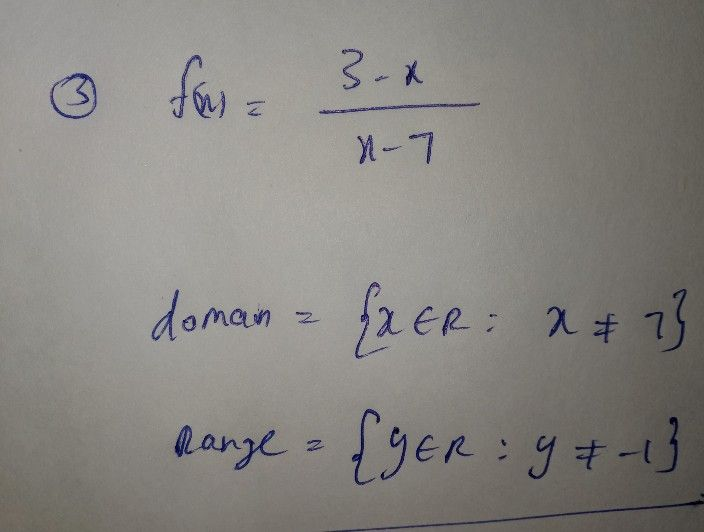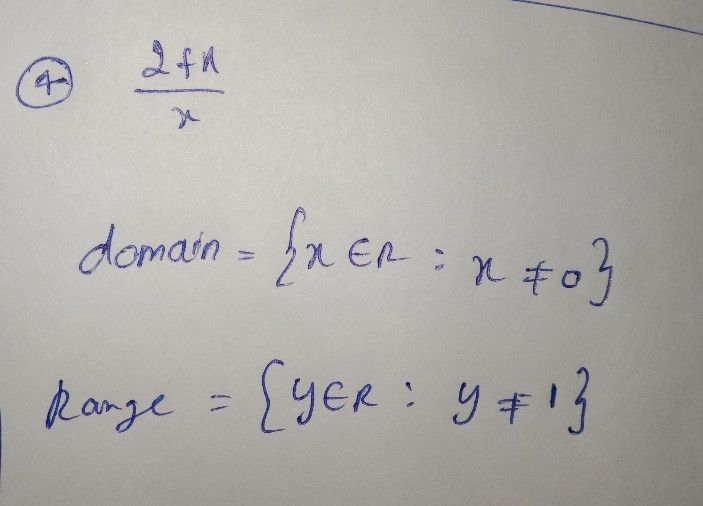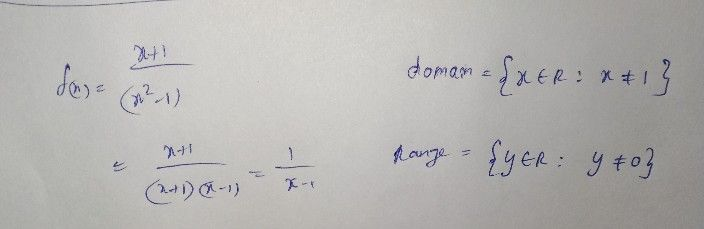hope you understood the answer
if you have any doubts feel free to ask meStudent
Hi ma'am,why the x in the denominator became nQanda teacher - Sneha
can please say which question you have doubt in so that I can explain once again clearly